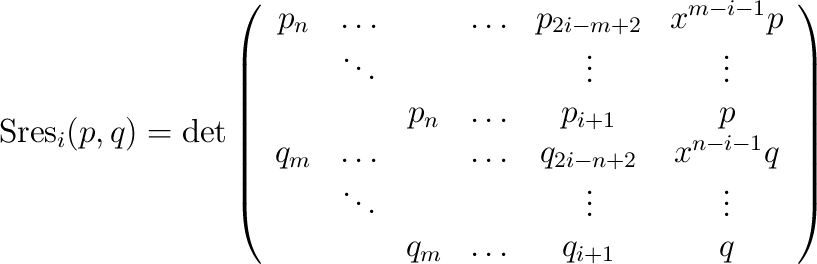## PolynomialTraits_d::PolynomialSubresultants

Note: This functor is optional!

### Definition

Computes the polynomial subresultant of two polynomials p and q of type PolynomialTraits_d::Polynomial_d with respect to outermost variable. Let p= i=0, ,np i xi and q= i=0, ,mq i xi, where x is the outermost variable. The i-th subresultant (with i=0, ,min {n,m}) is defined bywhere pi and qi are set to zero if i<0. In the case that n=m, Sresn is set to q.

The result is written in an output range, starting with the 0-th subresultant Sres0(p,q) (aka as the resultant of p and q).

### Operations

 template OutputIterator fo ( Polynomial_d p , Polynomial_d q , OutputIterator out ) computes the polynomial subresultants of p and q, with respect to the outermost variable. Each element is of type PolynomialTraits_d::Polynomial_d. template OutputIterator fo ( Polynomial_d p , Polynomial_d q , OutputIterator out , int i ) computes the polynomial subresultants of p and q, with respect to the variable xi.